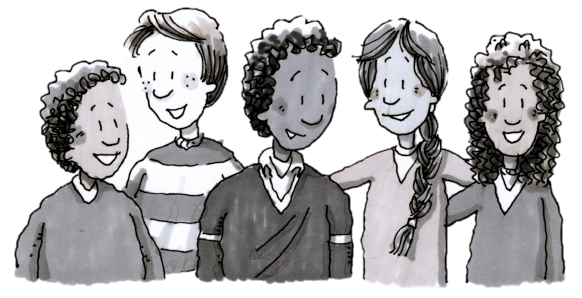### Home > CC2MN > Chapter 9 > Lesson 9.1.2 > Problem9-20

9-20.

There are five students of different ages. Their median age is $13$ years.

1. What are two possibilities for the ages of the three oldest students?

The median is the middle number of a data set ordered from least to greatest.

If $13$ is the median age in a set of five students, the two oldest students must be older than $13$.

2. If each student is a different age, how many students must be younger than $13$?

$\text{ __ __ } \ \color{blue}{13}\ \ \text{__ __}$

$2$ students

3. In complete sentences, describe what a median is to another student in the class. Pretend that the student was absent the day you learned about medians

See part (a).
What do you do when two numbers share the middle?